# PostgreSQL Tutorial: MIN Function: Get Minimum Value In a Set

August 3, 2023

Summary: in this tutorial, you will learn how to use PostgreSQL `MIN()` function to get the minimum value of a set.

## Introduction to PostgreSQL MIN function

PostgreSQL `MIN()` function an aggregate function that returns the minimum value in a set of values.

To find the minimum value in a column of a table, you pass the name of the column to the `MIN()` function. The data type of the column can be number, string, or any comparable type.

The syntax of the `MIN()` function is as follows:

``````SELECT
MIN(expression)
FROM
table_expression
...;
``````

Unlike the `AVG()`, `COUNT()` and `SUM()` functions, the `DISTINCT` option does not have any effects on the `MIN()` function.

## PostgreSQL MIN() function examples

We will use the `film` , `film_category`, and `category` tables from the dvdrental sample database for demonstration.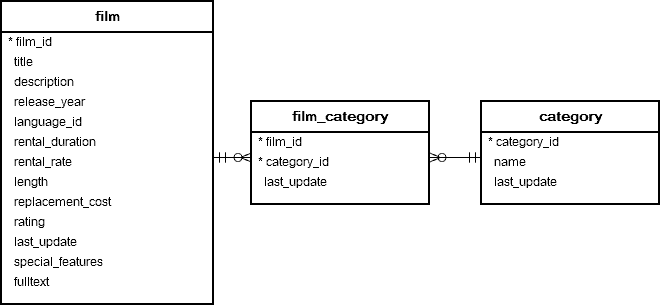### 1) Using PostgreSQL MIN function in SELECT clause

The following example uses the `MIN()` function to get the lowest rental rate from the `rental_rate` column the `film` table:

``````SELECT
MIN (rental_rate)
FROM
film;
``````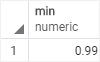The query returns 0.99, which is the lowest rental rate.

### 2) Using PostgreSQL MIN function in a subquery

To get films which have the lowest rental rate, you use the following query:

``````SELECT
film_id,
title,
rental_rate
FROM
film
WHERE
rental_rate = (
SELECT MIN(rental_rate)
FROM film
);
``````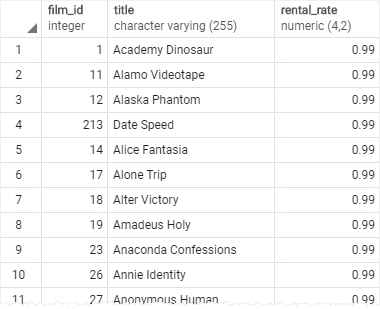How it works.

• First, the subquery to select the lowest rental rate.
• Then, the outer query selects films that have rental rates equal to the lowest rental rate returned by the subquery.

### 3) Using PostgreSQL MIN function with GROUP BY clause

In practice, you often use the `MIN` function with the `GROUP BY` clause to find the lowest value in each group.

The following statement uses the `MIN()` function with the `GROUP BY` clause to find the lowest replacement cost of films by category:

``````SELECT
name category,
MIN(replacement_cost) replacement_cost
FROM category
INNER JOIN film_category USING (category_id)
INNER JOIN film USING (film_id)
GROUP BY name
ORDER BY name;
``````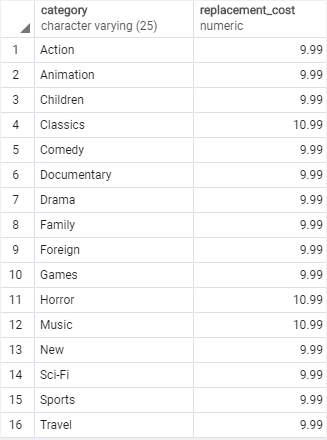### 4) Using PostgreSQL MIN function with HAVING clause

It’s possible to use the `MIN` function in the `HAVING` clause the filter the groups whose minimum values match a certain condition.

The following query finds uses the `MIN()` function to find the lowest replacement costs of films grouped by category and selects only groups that have replacement cost greater than `9.99`.

``````SELECT
name category,
MIN(replacement_cost) replacement_cost
FROM category
INNER JOIN film_category USING (category_id)
INNER JOIN film USING (film_id)
GROUP BY name
HAVING MIN(replacement_cost) > 9.99
ORDER BY name;
``````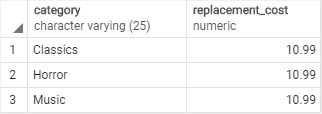### 5) Using PostgreSQL MIN function with other aggregate functions

It’s possible to use the `MIN()` function with other aggregate functions such as `MAX()` function in the same query.

The following example uses the `MIN()` and `MAX()` function to find the shortest and longest films by category:

``````SELECT
name category,
MIN(length) min_length,
MAX(length) max_length
FROM category
INNER JOIN film_category USING (category_id)
INNER JOIN film USING (film_id)
GROUP BY name
ORDER BY name;
``````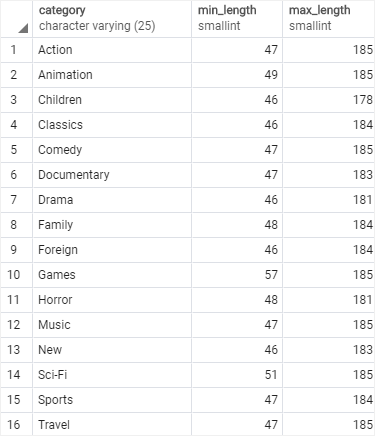## 6) Finding the smallest values from two or more columns

Suppose, you have the following `ranks` table:

``````CREATE TABLE ranks (
user_id INT PRIMARY KEY,
rank_1 int4 NOT NULL,
rank_2 int4 NOT NULL,
rank_3 int4 NOT NULL
);
``````

And its sample data:

``````INSERT INTO ranks
VALUES
(1, 6, 3, 5),
(2, 2, 8, 5),
(3, 5, 9, 8);
``````

Suppose you need to find the smallest rank for each user: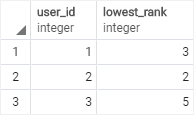In this case, you cannot use the `MIN()` function because the `MIN()` function is applied to rows, not columns. To find the minimum value of two or more columns, you use the `LEAST()` function:

``````SELECT
user_id,
LEAST (rank_1, rank_2, rank_3) AS lowest_rank
FROM
ranks;
``````

The query returns the result as we expected.

### Summary

• Use the `MIN()` function to find the lowest value in a set of values.
• Use the `MIN()` with `GROUP BY` clause to find the lowest value in a group of values.
• Use the `LEAST()` function to find minimum values between columns.